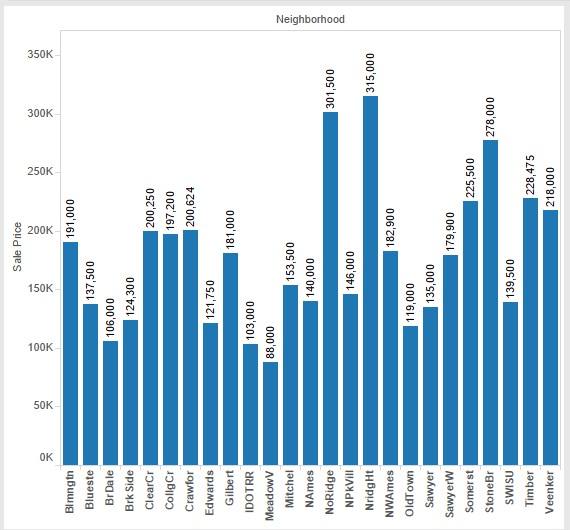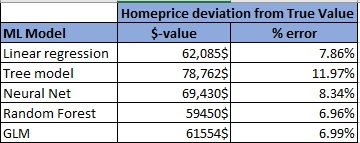Hello All,

In today’s tutorial we will apply 5 different machine learning algorithms to predict house sale prices using the Ames Housing Data.

This dataset is also available as an active Kaggle competition for the next month, so you can use this as a Kaggle starter script (in R). Use the output from the models to generate submission files for the Kaggle platform and view how well you fare on the public leaderboard.  This is also a perfect simulation for real-world analytics problem where the final results are validated by a customer / client/ third-party.

This tutorial is divided into 4 parts:

• Feature Selection
• Apply algorithms
• Using arithmetic / geometric means from multiple algorithms to increase accuracy

# Problem Statement:

Before we begin, let us understand the problem statement :

Predict Home SalePrice for the Test Data Set with the lowest possible error.

The Kaggle competition evaluates the leaderboard score based on the  Root-Mean-Squared-Error (RMSE)  between the logarithm of the predicted value and the logarithm of the observed sales price. So the best model will be one with a score of 0.

The evaluation considers log values so model is penalized for incorrectly predicting both expensive houses AND cheap houses. So the % of error deviation from real value matters, not just the \$ value of the homes.
E.g.: if the predicted value of homeprice was 42000\$ but actual value was 37000\$ , then \$-value error is only 5000\$ which doesn’t seem a lot. However, error % is ~13.52% .
On the contrary, imagine a home with real saleprice = 389411\$ , which we predicted to be 410000\$. \$ value difference is 20589\$, yet % error is only ~5.28% which is a better prediction.

In real-life too, you will frequently face situations where the sensitivity of predictions  is as important as value-accuracy.

As always,  you can download the code and data files from the Projects Page here , under the Feb 2017.

In this step we perform the following tasks:

1. Load the test and training set.
2. Check training set for missing values.
3. Delete columns with more than 40% missing records. These include the variables Alley (1369 empty ), FireplaceQu (690), Fence (1179), PoolQC (1453), MiscFeature (1406) .
4. The other variables identified in step 1  are:
• LotFrontage , Alley , MasVnrType, BsmtQual , BsmtCond , BsmtExposure , BsmtFinType1, BsmtFinType2,  GarageType , GarageCond.
5. For columns with very few missing values, we choose one of 3 options:
• For categorical values, create a new level “unk” to indicate missing data.
• For columns (numeric or categorical) where the data tends to fall overwhelmingly in one category , mark missing values with this option.
• For numeric data, mark missing values with -1.
• NOTE, we will apply these rules on the test set also , for consistency, irrespective of whether there are missing values or not.
6. Repeat steps 2-5 for test set columns, since there may be empty cells for columns in test set which did not show up in the training set.  The variables we now identify include:
• MSZoning, Utilities, Exterior1st, Exterior2nd, MasVnrArea,
• BsmtFinSF1, BsmtFinSF2, BsmtUnfSF, TotalBsmtSF.
• BsmtFullBath. BsmtHalfBath, KitchenQual,
• GarageYrBlt, GarageQual, GarageFinish, GarageCars, GarageArea,
• SaleType.
7. We write the modified training and test sets to Excel so we can apply our models on the corrected data. ( This is especially helpful in real life if you need to fine tune your model over a few days time.

# Feature Selection:

The easiest way to check if a relationship exists is to use statistical functions: chisquare, anova or correlation.

Our target variable is “SalePrice” (numeric value) . So we will test all the other predictor variables against this factor to see if any relation exists and how much it affects the SalePrice.
For categorical predictors, we will use chisquare test, whereas for numeric predictors, we will use correlation.

Our dataset has 74 predictive factors (excluding SalePrice and Id), so we run a for loop to do a rough check. Relation exists only if p-values < 0.05.
If the predictor column is of type integer/numeric, we will apply correlation. If column = “character” , we apply chisquare.

We also add a column to identify variables of interest using the code below:

• If correlation value falls below -0.75 (high negative correlation) or above 0.75 (high positive correlation) then we mark the variable as “match”.
• If p-val from chisquare test falls below 0.05 then we mark it as “match”.

Using this “quack” approach, we quickly identify 19 variables of interest. These include Neighborhood, Building Type (single family, townhome, etc) , YearBuilt, Year Remodeled , Basement type (finished / unfinished), house area for first floor/ second floor / garage, number of bathrooms (both full / half) , no of cars garage can accommodate, sale type and sale condition.

NOTE, it is always a good idea to graphically visualize correlation functions as the above approach may miss out predictors with a non-linear relationship.Median House SalePrice by Neighborhood

# Apply Algorithms:

We apply the following machine learning algorithms:

1. Linear Regression Model.
2. Classification Tree Model.
3. Neural Network Model.
4. Random Forest algorithm model.
5. Generalized Linear Model (GLM)

We follow the same steps for all 5 models:
(Note, code and functions shown only for Linear Regression Model. Detailed functions and variables used for the other models are available in the R program files.)

1. Use the training set to create a formula.
2. Apply formula to predict values for validation set.
3. Check the deviation from true homeprices in terms of both median \$-value and % error.
4. Apply formula on test set and check rank/score on leaderboard.

The error rates for all 5 models are given in the table below:home price table showing model error %

# Combining Algorithms to Improve Accuracy:

There are many scientific papers which show that combining answers from multiple models greatly improves accuracy. A simple but excellent explanation (with respect to Kaggle ) is given by MLwave.com founder and past Kaggle winner is  provided here. Basically, combining results from unrelated models can improve accuracy, even if individual models are NOT good at 100% accurate predictions.

Similar to the explanation provided in the link, we calculate the arithmetic mean to average the results from all 5 models .

# Summary:

We learnt how to clean and process a real-life dataset, select features of interest (and impact on target variable) apply 5 different machine learning algorithms.

The code for this tutorial is available here on the Projects page, under month of Feb.

Please take a look and feel free to comment with your own feedback or models.